# 区间调度之区间交集问题

## 区间调度之区间交集问题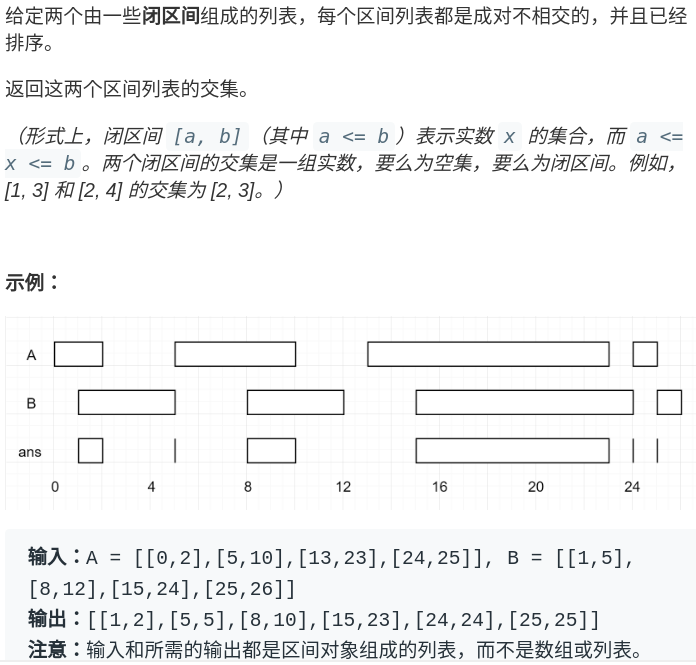# A, B 形如 [[0,2],[5,10]...]
def intervalIntersection(A, B):
i, j = 0, 0
res = []
while i < len(A) and j < len(B):
# ...
j += 1
i += 1
return res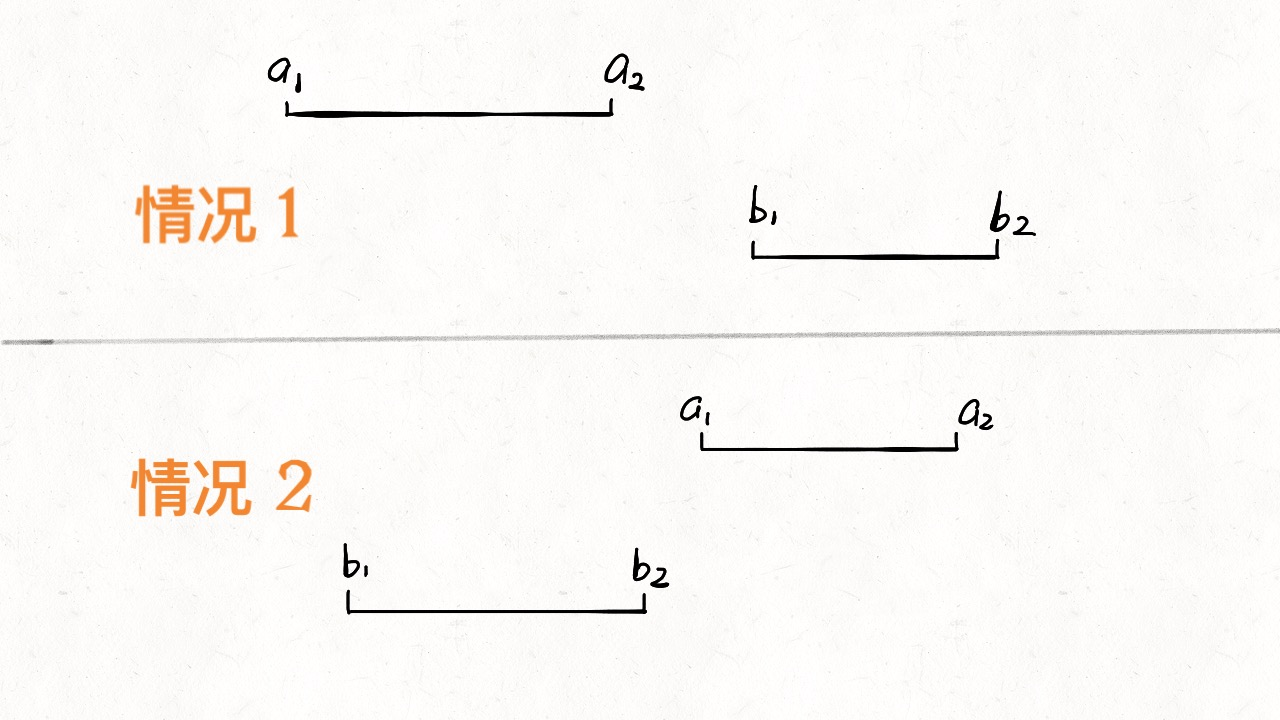if b2 < a1 or a2 < b1:
[a1,a2] 和 [b1,b2] 无交集


# 不等号取反，or 也要变成 and
if b2 >= a1 and a2 >= b1:
[a1,a2] 和 [b1,b2] 存在交集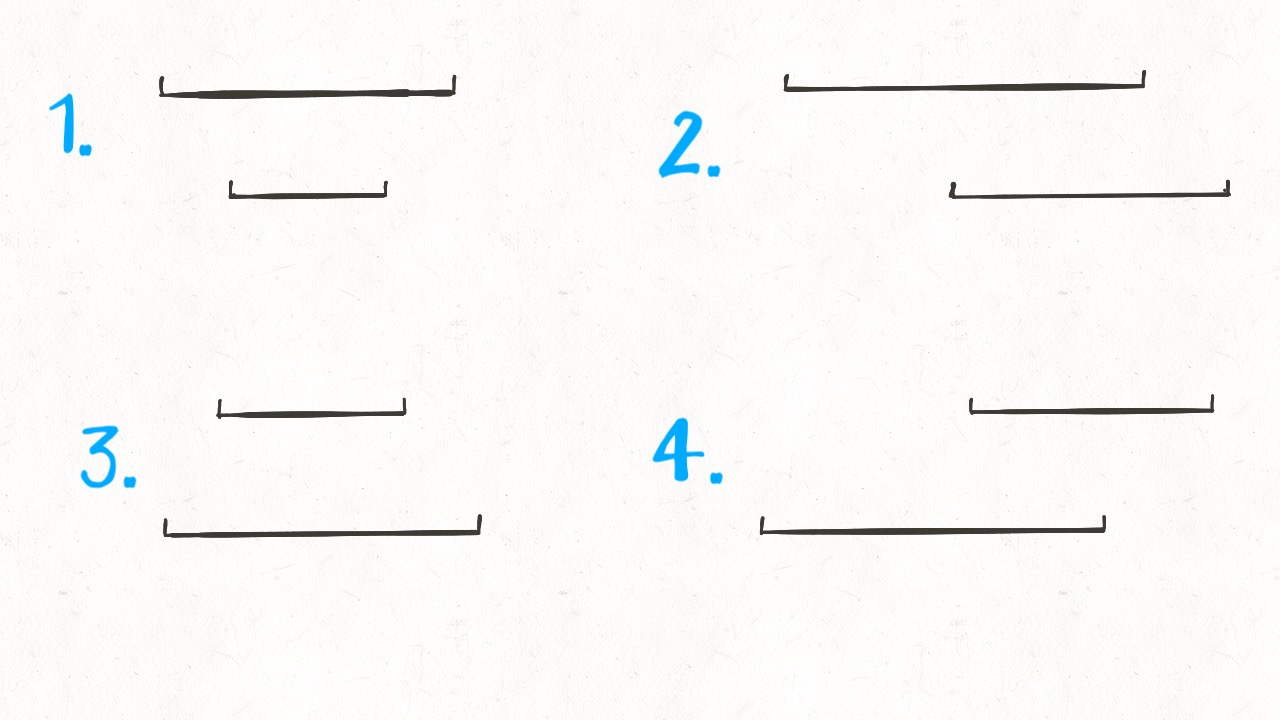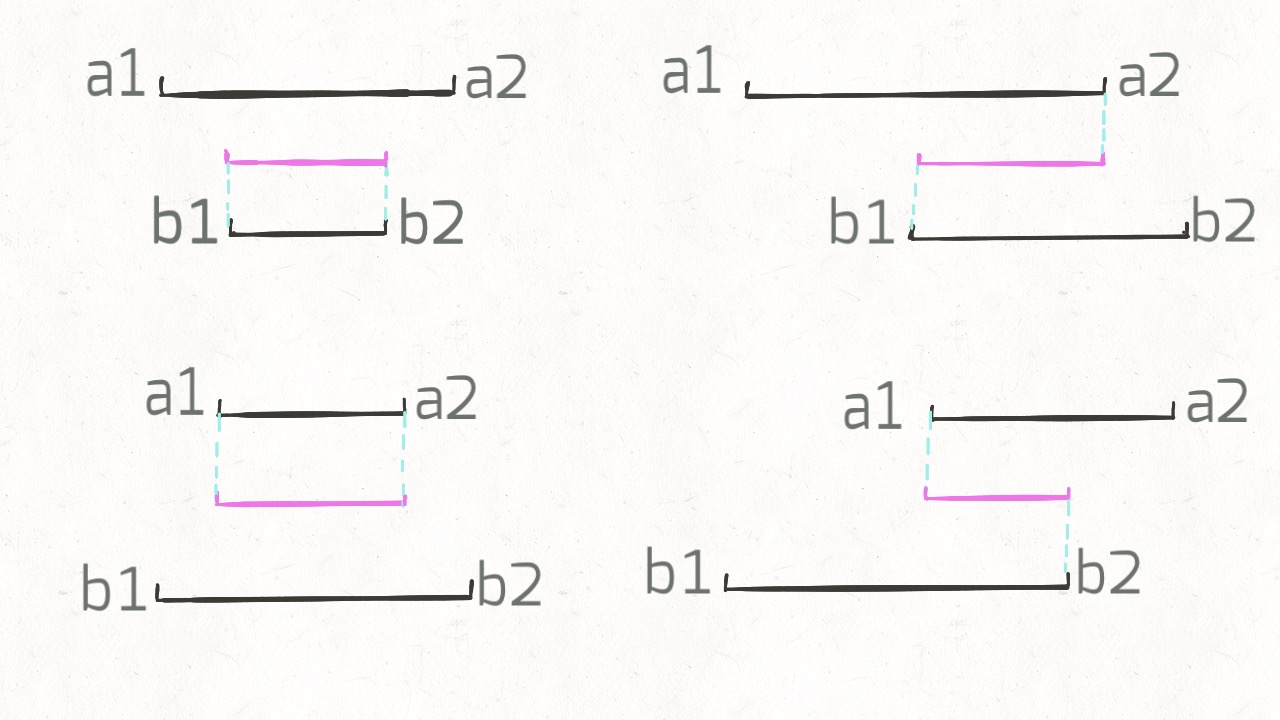while i < len(A) and j < len(B):
a1, a2 = A[i], A[i]
b1, b2 = B[j], B[j]
if b2 >= a1 and a2 >= b1:
res.append([max(a1, b1), min(a2, b2)])
# ...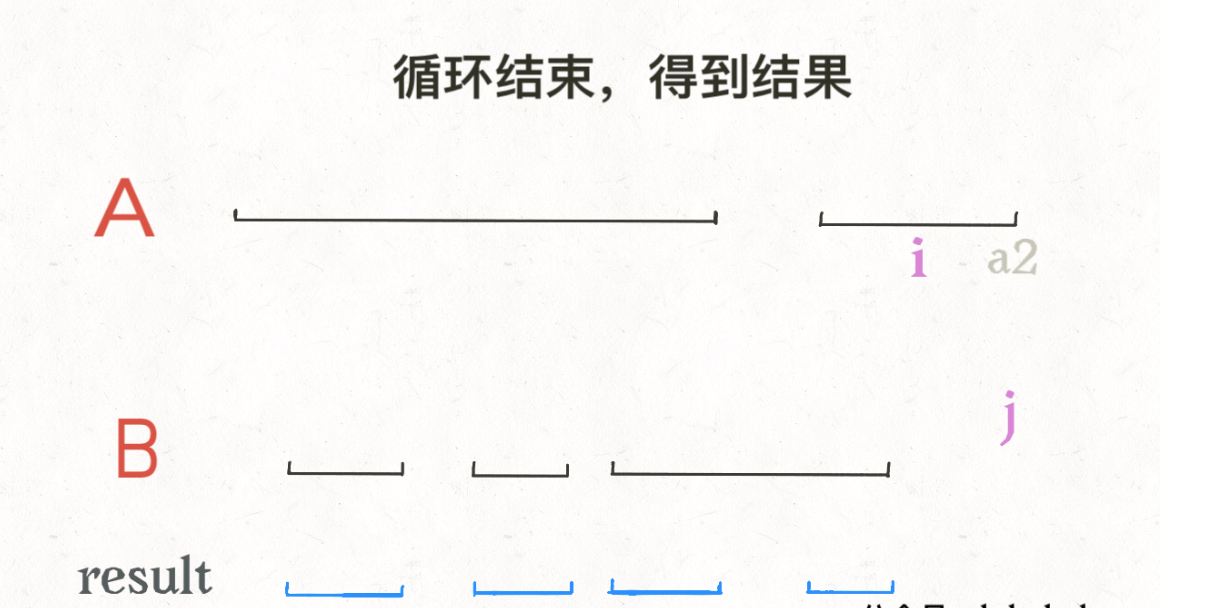while i < len(A) and j < len(B):
# ...
if b2 < a2:
j += 1
else:
i += 1


# A, B 形如 [[0,2],[5,10]...]
def intervalIntersection(A, B):
i, j = 0, 0 # 双指针
res = []
while i < len(A) and j < len(B):
a1, a2 = A[i], A[i]
b1, b2 = B[j], B[j]
# 两个区间存在交集
if b2 >= a1 and a2 >= b1:
# 计算出交集，加入 res
res.append([max(a1, b1), min(a2, b2)])
# 指针前进
if b2 < a2: j += 1
else:       i += 1
return res


C++代码：

class Solution {
public:
vector<vector<int>> intervalIntersection(vector<vector<int>>& A, vector<vector<int>>& B) {
//保存结果
vector<vector<int>> ret;
if(A.empty() || B.empty())
{
return ret;
}

//i和j两个下标索引
int i = 0;
int j = 0;
while(i < A.size() && j < B.size())
{
//下面四个变量的含义相见博客
int a1 = A[i];
int a2 = A[i];
int b1 = B[j];
int b2 = B[j];

//代表区间有交集，自己画个图就OK
if(b2 >= a1 && a2 >= b1)
{
//max(a1,b1),min(a2,b2)两个区间的交集部分
ret.push_back({max(a1,b1),min(a2,b2)});
}

//更新i和j下标索引，b2 < a2 就代表i所在的区间大于j所在的区间，更新j
//是因为i区间长于j区间的部分还可能和j的下一个区间继续重叠，还需要继续判断
//所以更新j而不是更新i
if(b2 < a2)
{
j++;
}
else//反之同理
{
i++;
}
}
return ret;
}
};


01-1458408-153959
02-03699
12-29362
06-05192
01-11277
03-16871
08-15340
03-07390
09-121088
12-2330
07-02372
01-08291
05-0818
07-15189
11-2822
11-1216
09-2557
01-18243
©️2020 CSDN 皮肤主题: 编程工作室 设计师:CSDN官方博客